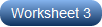# Convert between Decimals and Octals Worksheets

Unlike the decimal number system that incorporates base 10 numerals, the octal number system uses the numerals 0 to 7 leaving off the digits 8 and 9. This means a number in the decimal system has an equivalent octal representation while a number in the octal system gets a decimal counterpart. Gain adequate practice of the various algorithms and strategies used in decimal to octal conversion and octal to decimal conversion with this set of printable high school worksheets. Access one of these handouts for free!

Convert Decimals to Octals

Master converting base-10 to base-8 numerals! Divide numbers by 8 until you get a quotient < 8; frame the octal equivalents by listing the remainders obtained on every step backward.